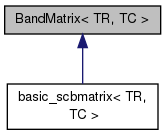CVM Class Library  8.1 This C++ class library encapsulates concepts of vector and different matrices including square, band, symmetric and hermitian ones in Euclidean space of real and complex numbers.
BandMatrix< TR, TC > Class Template Reference

Generalized band matrix class (not end-user) More...

#include <cvm.h>

Inheritance diagram for BandMatrix< TR, TC >:[legend]

Public Member Functions

tint lsize () const
Number of lower sub-diagonals.
tint usize () const
Number of upper super-diagonals.

Protected Member Functions

BandMatrix ()
internal protected constructor
BandMatrix (tint nKL, tint nKU)
internal protected constructor
virtual ~BandMatrix ()
Destructor.

Protected Attributes

tint mkl
Number of sub-diagonals.
tint mku
Number of super-diagonals.

Detailed Description

template<typename TR, typename TC> class BandMatrix< TR, TC >

Generalized band matrix class (not end-user)

TR type stands for treal, TC type stands for tcomplex. Please use inherited band square matrix classes. This one provides some member functions which are common for all band square matrices. Way of elements storage:

L=1, U=2 L=2, U=3 L=0, U=0 L=1, U=0
- - d d
-- -- d *d
d** --- d *d
*d** d*** d *d
*d** *d*** d *d
*d** **d*** d *d
*d** **d** d _
*d* **d*
*d **d
- --
-
Matrix

Definition at line 27811 of file cvm.h.

Constructor & Destructor Documentation

template<typename TR, typename TC>
 BandMatrix< TR, TC >::BandMatrix ( )
inlineprotected

internal protected constructor

Definition at line 27818 of file cvm.h.

template<typename TR, typename TC>
 BandMatrix< TR, TC >::BandMatrix ( tint nKL, tint nKU )
inlineprotected

internal protected constructor

Definition at line 27825 of file cvm.h.

template<typename TR, typename TC>
 virtual BandMatrix< TR, TC >::~BandMatrix ( )
inlineprotectedvirtual

Destructor.

Definition at line 27832 of file cvm.h.

Member Function Documentation

template<typename TR, typename TC>
 tint BandMatrix< TR, TC >::lsize ( ) const
inline

Number of lower sub-diagonals.

Returns number of lower sub-diagonals of calling band matrix.

Example:
using namespace cvm;
srbmatrix m(4,2,1);
m.set(1.);
std::cout << m;
std::cout << m.msize() << " " << m.nsize() << " " << m.size();
std::cout << " " << m.lsize() << " " << m.usize() << std::endl;
prints
1 1 0 0
1 1 1 0
1 1 1 1
0 1 1 1
4 4 16 2 1
Returns
Result value.

Definition at line 28237 of file cvm.h.

Here is the caller graph for this function:template<typename TR, typename TC>
 tint BandMatrix< TR, TC >::usize ( ) const
inline

Number of upper super-diagonals.

Returns number of upper super-diagonals of calling band matrix.

Example:
using namespace cvm;
srbmatrix m(4,2,1);
m.set(1.);
std::cout << m;
std::cout << m.msize() << " " << m.nsize() << " " << m.size();
std::cout << " " << m.lsize() << " " << m.usize() << std::endl;
prints
1 1 0 0
1 1 1 0
1 1 1 1
0 1 1 1
4 4 16 2 1
Returns
Result value.

Definition at line 28264 of file cvm.h.

Here is the caller graph for this function:Member Data Documentation

template<typename TR, typename TC>
 tint BandMatrix< TR, TC >::mkl
protected

Number of sub-diagonals.

Definition at line 27814 of file cvm.h.

template<typename TR, typename TC>
 tint BandMatrix< TR, TC >::mku
protected

Number of super-diagonals.

Definition at line 27815 of file cvm.h.

The documentation for this class was generated from the following file: